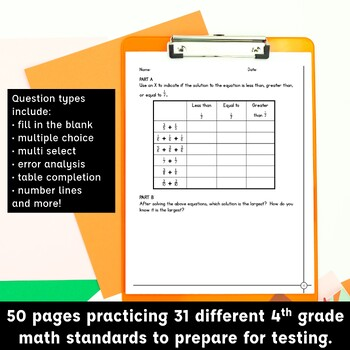# 4th Grade Math Performance Based Assessments Practice PrintablesSubjects
Resource Type
Formats Included
Zip (2 MB|52 pages)
Standards
\$10.00
\$10.00

### Description

Prepare your 4th grade students for the rigors of end of year math state tests like SBAC and PARCC with these practice printables. These days standardized tests are so complex and rigorous that students need exposure to test formats and question types in order to be successful at showing their mastery of the grade level standards.

These practice printables were designed to give students practice and exposure with a variety of question types similar to what they will see on the PARCC or SBAC. Every problem is set up in two parts with students working through multi-step problems in a variety of formats. It's intentionally designed to be rigorous and well-aligned to the standards.

Question types include:
• fill in the blank
• multiple choice
• multiple choice with multiple correct answers
• error analysis
• table completion
• completing number lines
and more!

The preview offers a look at several pages in the unit giving you a glimpse of standards covered and question types.

While no one enjoys test-prep and it should not be a major focus, and daily instruction is the ultimate key to student success, printable practice such as these can give students that extra exposure that makes them confident with their skills.
Total Pages
52 pages
Included
Teaching Duration
N/A
Report this Resource to TpT
Reported resources will be reviewed by our team. Report this resource to let us know if this resource violates TpT’s content guidelines.

### Standards

to see state-specific standards (only available in the US).
Compare two decimals to hundredths by reasoning about their size. Recognize that comparisons are valid only when the two decimals refer to the same whole. Record the results of comparisons with the symbols >, =, or <, and justify the conclusions, e.g., by using a visual model.
Use decimal notation for fractions with denominators 10 or 100. For example, rewrite 0.62 as 62/100; describe a length as 0.62 meters; locate 0.62 on a number line diagram.
Express a fraction with denominator 10 as an equivalent fraction with denominator 100, and use this technique to add two fractions with respective denominators 10 and 100. For example, express 3/10 as 30/100, and add 3/10 + 4/100 = 34/100.
Solve word problems involving multiplication of a fraction by a whole number, e.g., by using visual fraction models and equations to represent the problem. For example, if each person at a party will eat 3/8 of a pound of roast beef, and there will be 5 people at the party, how many pounds of roast beef will be needed? Between what two whole numbers does your answer lie?
Understand a multiple of 𝘢/𝘣 as a multiple of 1/𝘣, and use this understanding to multiply a fraction by a whole number. For example, use a visual fraction model to express 3 × (2/5) as 6 × (1/5), recognizing this product as 6/5. (In general, 𝘯 × (𝘢/𝘣) = (𝘯 × 𝘢)/𝘣.)